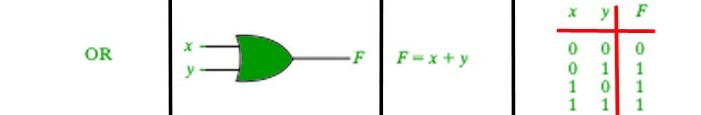# || operator in Java

• Last Updated : 01 Nov, 2020

|| is a type of Logical Operator and is read as “OR OR” or “Logical OR“. This operator is used to perform “logical OR” operation, i.e. the function similar to OR gate in digital electronics.Attention reader! Don’t stop learning now. Get hold of all the important Java Foundation and Collections concepts with the Fundamentals of Java and Java Collections Course at a student-friendly price and become industry ready. To complete your preparation from learning a language to DS Algo and many more,  please refer Complete Interview Preparation Course.

One thing to keep in mind is the second condition is not evaluated if the first one is true, i.e. it has a short-circuiting effect. Used extensively to test for several conditions for making a decision.
Syntax:

```Condition1 || Condition2

// returns true if one of the conditions is true.
```

Below is an example to demonstrate || operator:
Example:

## Java

 `// Java program to illustrate``// logical OR operator` `import` `java.util.*;` `public` `class` `operators {``    ``public` `static` `void` `main(String[] args)``    ``{` `        ``char` `ch = ``'a'``;` `        ``// check if character is alphabet or digit``        ``// using || operator``        ``if` `(ch >= ``65` `&& ch <= ``90``            ``|| ch >= ``97` `&& ch <= ``122``)``            ``System.out.println(``                ``ch``                ``+ ``" is an alphabet."``);``        ``else` `if` `(ch >= ``48` `&& ch <= ``57``)``            ``System.out.println(``                ``ch``                ``+ ``" is a digit."``);``        ``else``            ``System.out.println(``                ``ch``                ``+ ``" is a special character."``);``    ``}``}`
Output:
```a is an alphabet.

```

My Personal Notes arrow_drop_up